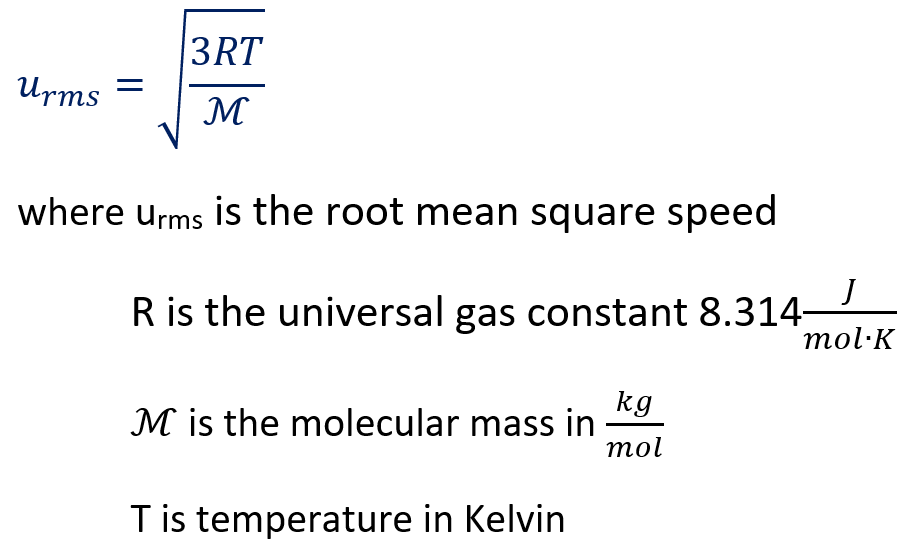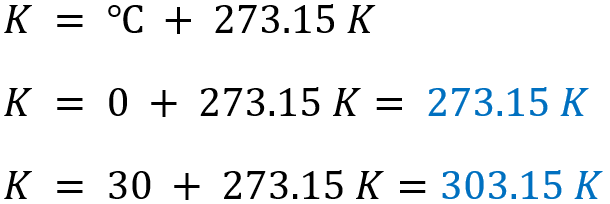# Problem: Helium (He) is the lightest noble gas component of air, and xenon (Xe) is the heaviest. [For this problem, use R = 8.314 J/(mol·K) and express ℳ in kg/mol.](a) Find the rms speed of He in winter (0.°C) and in summer (30.°C).

###### FREE Expert Solution

The formula to calculate the room mean square speed is:Since the given temperature is in °C, we need to convert it into Kelvin.97% (256 ratings)###### Problem Details

Helium (He) is the lightest noble gas component of air, and xenon (Xe) is the heaviest. [For this problem, use R = 8.314 J/(mol·K) and express ℳ in kg/mol.]

(a) Find the rms speed of He in winter (0.°C) and in summer (30.°C).